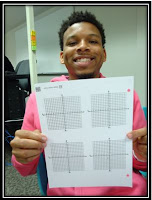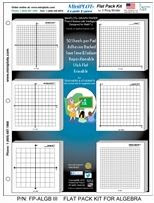### Tools for Helping Students Graph Equations

I work in the math lab at the community college where I also teach. The math lab is staffed by only math instructors and offers free math tutoring to any of our students. We try to have many resources available for our students. When it comes to graphing, we have found that the computer can be very unfriendly. The graphs are often hard to see, and so finding points is next to impossible. We keep in stock some items that help our students.First, we have graph paper that is always available. We keep an assortment of different kinds for our students:
1. 1/4" grid paper
2. Four co-ordinate graphs per page
3. Full co-ordinate graph paper
4. Six small co-ordinate graphs per page
On the right, you see Markwan holding the example of #2.

We also have two-sided white boards. One side is blank while the opposite side contains a coordinate graph outline. Our students make good use of these. They like the fact that they can do the linear or quadratic equation on one side and then construct the graph on the other.  (They don't have to draw the X-Y axes and tick marks for each problem or get out the ruler for accuracy.) Since the white boards are erasable, they can be used over and over again. On the left, Sam is "modeling" the white board. (Both young men wanted to be on my blog and were anxious for me to use their first names.)Click Here for More Information
BUT my favorite item we have on hand are graphing sticky notes.  I often use them in my math classes because students can take notes while drawing examples of graphs and then stick the example right into their math book.  These post-it-notes are called MiniPLOTs®.  They are a unique brand of Post-It Notes designed for math students, teachers and tutors. MiniPLOTs® are 3x3" paper pads with 50 coordinate grid, polar coordinates, or 3D solid shapes printed on each sheet. They are the perfect size for homework and tests. In addition, the company makes them for algebra, geometry, trigonometry, statistics and K-6 math (provides an innovative method of teaching students the basic multiplication and division factors in about six weeks).

These work great when I am grading math homework. When a student has graphed an equation wrong, I simply take a graphing sticky note, correctly graph the equation and stick it beside their incorrect answer. It's important that students see the correct answer so that the wrong one doesn't remain stuck in their heads!

The Math Lab also supplies a reference sheet entitled Graphs of Some Common Functions. It gives an example of the equation being graphed (i.e. f(x) = x ), a visual of the what the graph should look like, the domain, range, and symmetry origin. The students are free to use the laminated ones in the Math Lab, but can also take home a paper one to place in their math notebooks.

On the reverse side of this reference sheet are examples of: Graph of f(x) = ax and Graph of f(x) = loga(x). Besides a visual of the graph, it includes domain, range, decreasing on and horizontal or vertical asymptote.

Most students are visual learners and can see lines and curves and project how they behave intuitively. Their brains can easily understand, understand and recognize pictorially better than just remembering abstract equations.  It is therefore important for students to construct and draw graphs so they can picture them in their minds. Hopefully some of these graphing tools will make constructing those graphs easier.

### Using Free Milk Lid Jug Lids for Hands-On Math ActivitiesMilk Lid Math
Start saving milk jug lids because there are countless hands-on math activities you can do in your classroom using this free manipulative. Here are just four of those ideas.

1) Sort the lids by various attributes such as:
• Color
• Snap-on or Twist-on
• Label or No Label
• Kind of edge (smooth or rough)
2) Let the students grab one handful of lids.
• Ask the students to count the lids.
• See if the students can write that number.
3) Make a pattern using two different colors of lids.

• Identify the pattern using letters of the alphabet or numbers. The pattern above would be an A, A, B pattern or a 1, 1, 2 pattern.
• Now ask the students to use more than two colors to make a pattern
• Once more, have the students identify the pattern using alphabet letters or numbers.
4) Decide on a money value for each color of lid. (Example: Red lids are worth a nickel, blue lids are worth a dime, and white lids are worth a penny.) Put all of the lids into a bag and have the students draw out four lids. Have the students add up the total value of these four lids.
•\$3.00
Use play money (coins) to have the students show the value of the lids.
• Have the students practice writing money as either a part of a dollar or as cents.
• Another idea is to have the students find all the combinations of lids that would equal a nickel or a dime or a quarter.
The resource, Milk Lid Math, contains over 15 hands-on ideas with numerous activities listed under each one. These may be used with a whole group, small groups or as center activities.

### The ROOT of the Problem - What is Digital Root and How to Use It

When students skip count, they can easily say the 2's, 5's, and 10's which translates into easy memorization of those particular multiplication facts.  Think what would happen if every primary teacher had their students practice skip counting by 3's, 4's, 6's, 7's, 8's and 9's!  We would eradicate the drill and kill of memorizing multiplication and division facts.

Since many of my college students do not know their facts, I gravitate to the Divisibility Rules.  Sadly, most have never seen or heard of them.  I always begin with dividing by 2 since even numbers are understood by almost everyone.  (Never assume a student knows what an even number is as I once had a college student who thought that every digit of a number must be even for the entire number to be even.) We then proceed to the rules for 5 and 10 as most students can skip count by those two numbers.

Finally, we learn about the digital root for 3, 6, and 9. This is a new concept but quickly learned and understood by the majority of my students. (See the definition below).

Here are several examples of finding Digital Root:

1) 123 = 1 + 2 + 3 = 6. Six is the digital root for the number 123. Since 123 is an odd number, it is not divisible by 6. However, it is still divisible by 3.

2) 132 = 1 + 3 + 2 = 6. Six is the digital root for the number 132. Since 132 is an even number, it is divisible by 6 and by 3.

3) 198 = 1+ 9 + 8 = 18 = 1 + 8 = 9. Nine is the digital root for the number 198; so, 198 is divisible by 9 as well as by 3.

4) 201 = 2 + 0 + 1 = 3. Three is the digital root for the number 201; so, 201 is divisible by 3.

The first time I learned about Digital Root was about eight years ago at a workshop presented by Kim Sutton. (If you have never been to one of her workshops - GO! It is well worth your time.) Anyway, I was beside myself to think I had never learned Digital Root. Oh, the math classes I sat through, and the numbers I tried to divide by are too numerous to mention! It actually gives me a mathematical headache. And to think, not knowing Digital Root was the ROOT of my problem!

### Using Lattice Multiplication in College - A Good or Bad Idea?

I work in the math lab at the college where we teach. All of our students have free access to this tutoring. This is one of my favorite things to do since I am working mostly one-on-one with students. Last week, I had a student who is taking Fundamentals of Algebra. It is a remedial class for those who do not pass the math test to take College Algebra. In this particular class, students are not allowed to use calculators. In other words, it helps if you know your math facts (and sadly, many don’t).

She had to multiply a three-digit number by a two-digit number in one of her word problems. Pretty easy, right? She kept telling me that she could only multiply using the “lettuce” form of multiplication. Many tutors in the lab had no idea what she was talking about; so, I asked her, “Do you mean lattice multiplication?” Once she demonstrated it to the group, I knew that she was using lattice multiplication.

For those of you unfamiliar with it, here is an illustration of what it looks like.
Step #1

Draw a grid so that each digit has its own box. If it is a 3-digit by 3-digit problem, you will need a grid that is three by three. If it is a 3-digit problem by a 2-digit problem, you will need a grid that is three by two.

Step #2

Write the digits for the first factor going across the top of the lattice (grid), one digit per box. Write the second digits on the right going down the lattice, one number per box.

Step #3

Now, divide each box in half by drawing a diagonal line, starting in the top right corner and moving to the bottom left corner. Use a ruler if you like nice straight lines. You can have the diagonal lines continue so that they are outside of the grid boxes. This will help you with your answer!

Step #4

Work through the lattice and multiply each number together. Write the answers in the box. The number in the tens place goes in the upper part of the box, the number in the ones places goes in the lower part of the box. If there is not a number in the tens place, put a zero.

Step #5

To finish, you just add down the diagonal lines. Remember to regroup if necessary

Step #6

Finally, to figure out your product, read the numbers from the left of the grid around to the bottom of the grid.  In the example above, 789 x 461 = 363,729.

This form of multiplication dates back to the 1200s or before in Europe. It gets its name from the fact that to do the multiplication you fill in a grid which resembles a garden lattice, something you might find ivy growing on. Although it works, it is a pretty lengthy process for a college student, especially when she only has so much time to complete a test.

The “vertical” method of multiplication is more efficient because most can write down and solve a 3-digit by 3-digit multiplication problem faster than many students can even draw the lattice. Unfortunately, I showed this student several other ways to multiply, but she failed to grasp their significance. She said that is how she learned in fifth grade, and she was too old to change! With all of that said, lattice multiplication will make it easier for her to transition from lattice multiplication of whole numbers to multiplication of polynomials.